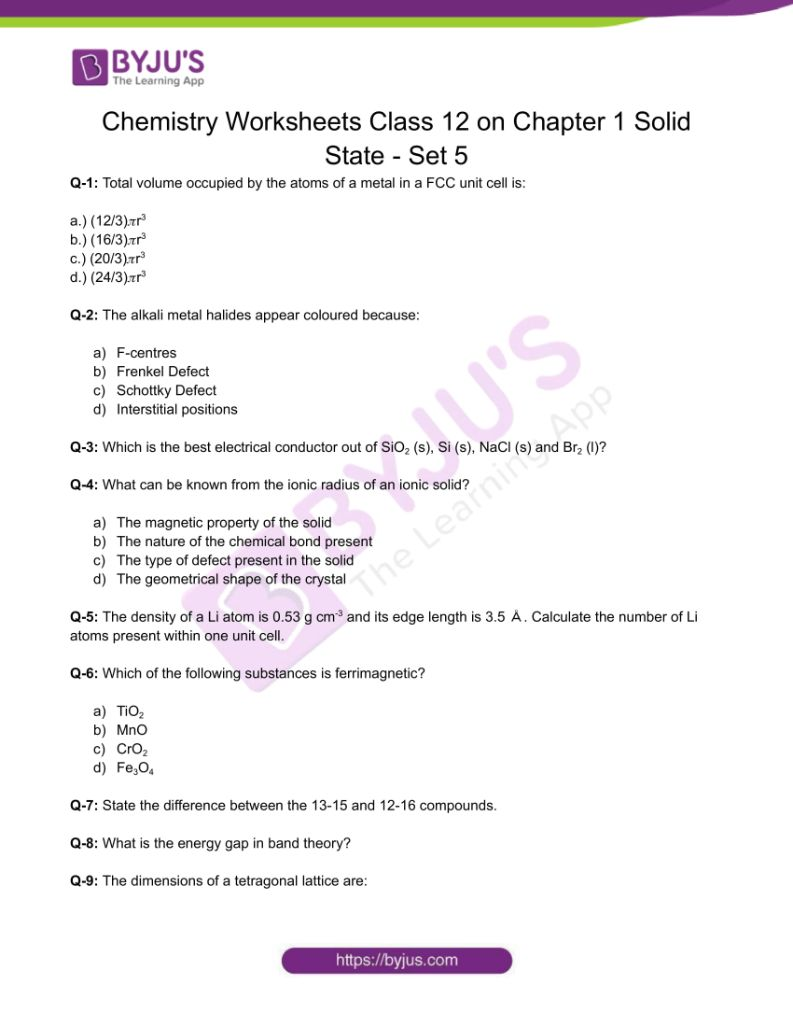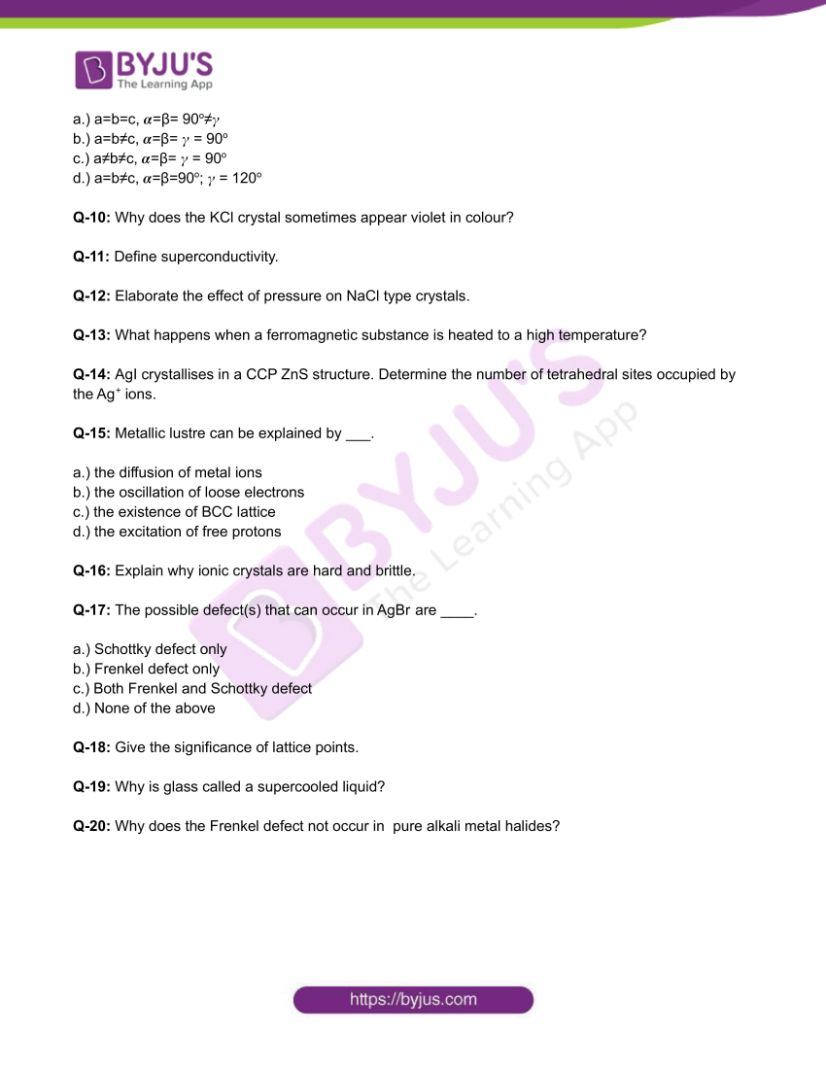Checkout JEE MAINS 2022 Question Paper Analysis : Checkout JEE MAINS 2022 Question Paper Analysis :

# Chemistry Worksheets Class 12 on Chapter 1 Solid State with Answers - Set 5

There are three states of all substances; out of which the solid state substances are most abundant. The crystalline solids have a long range order which implies that the particles are arranged in a regular manner in the three dimensional space. In amorphous solids, there is no regular arrangement of particles in the three dimensional space.

## 👇## CBSE Class 12 Chemistry Chapter 1 Solid State Worksheet – Set 5

Q-1: Total volume occupied by the atoms of a metal in a FCC unit cell is:

a.) (12/3)𝜋r3

b.) (16/3)𝜋r3

c.) (20/3)𝜋r3

d.) (24/3)𝜋r3

Q-2: The alkali metal halides appear coloured because:

a.) F-centres

b.) Frenkel Defect

c.) Schottky Defect

d.) Interstitial positions

Q-3: Which is the best electrical conductor out of SiO2 (s), Si (s), NaCl (s) and Br2 (l)?

Q-4: What can be known from the ionic radius of an ionic solid?

a.) The magnetic property of the solid

b.) The nature of the chemical bond present

c.) The type of defect present in the solid

d.) The geometrical shape of the crystal

Q-5: The density of a Li atom is 0.53 g cm-3 and its edge length is 3.5 Å. Calculate the number of Li atoms present within one unit cell.

Q-6: Which of the following substances is ferrimagnetic?

a.) TiO2

b.) MnO

c.) CrO2

d.) Fe3O4

Q-7: State the difference between the 13-15 and 12-16 compounds.

Q-8: What is the energy gap in band theory?

Q-9: The dimensions of a tetragonal lattice are:

a.) a=b=c, 𝜶=β= 90o≠𝛾

b.) a=b≠c, 𝜶=β= 𝛾 = 90o

c.) a≠b≠c, 𝜶=β= 𝛾 = 90o

d.) a=b≠c, 𝜶=β=90o; 𝛾 = 120o

Q-10: Why does the KCl crystal sometimes appear violet in colour?

Q-11: Define superconductivity.

Q-12: Elaborate the effect of pressure on NaCl type crystals.

Q-13: What happens when a ferromagnetic substance is heated to a high temperature?

Q-14: AgI crystallises in a CCP ZnS structure. Determine the number of tetrahedral sites occupied by the Ag+ ions.

Q-15: Metallic lustre can be explained by ___.

a.) the diffusion of metal ions

b.) the oscillation of loose electrons

c.) the existence of BCC lattice

d.) the excitation of free protons

Q-16: Explain why ionic crystals are hard and brittle.

Q-17: The possible defect(s) that can occur in AgBr are ____.

a.) Schottky defect only

b.) Frenkel defect only

c.) Both Frenkel and Schottky defect

d.) None of the above

Q-18: Give the significance of lattice points.

Q-19: Why is glass called a supercooled liquid?

Q-20: Why does the Frenkel defect not occur in pure alkali metal halides?

Download the PDF to access answers to the Chemistry Worksheet for Class 12 Chemistry Chapter 1 Solid State Set -5.